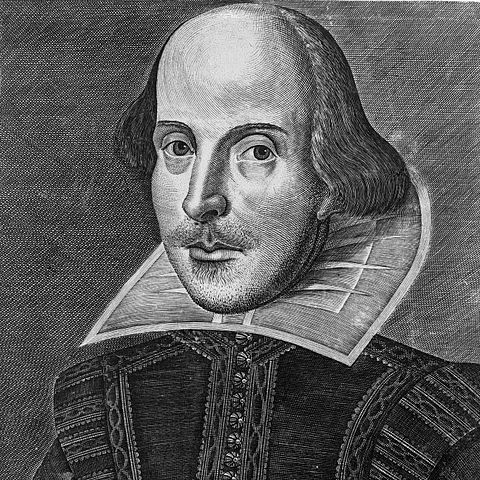## It ain't necessarily so!

We have strong feelings (Intuition) about what is true and not true

Intuition about the physical world is wrong

Special Relativity

Heisenberg Uncertainty

Quantum Entanglement

Stern Gerlach

## But this talk isn't about that

Mathematical Certainty

Using logic to derive conclusions

What does a Proof look like?

## Equivalence Relations

Reflexive: $a \simeq a$

Symmetric: $a \simeq b \Rightarrow b \simeq a$

Transitive: $a \simeq b \wedge b \simeq c \Rightarrow a \simeq c$

An equivalence relation induces a partition

The set R of real numbers: $a \simeq b \Leftrightarrow b - a$ is an rational number

## What is Length?

The length of an interval $\left (a, b \right )$ or $\left [a, b \right ]$ should be $b-a$

If you have two disjoint intervals the length of their union should be the sum of their lengths.

This extends to finitely many disjoint unions.

And even to infinitely many disjoint unions.

$\left [0,\frac{1}{2} \right ) \cup \left [\frac{1}{2}, \frac{3}{4} \right ) \cup \left [\frac{3}{4}, \frac{7}{8} \right ) ... = \left [0, 1 \right )$ has length 1

# Sets

A collection of objects: $\left \{ 1,2 \right \}$ or $\left \{a,b,c \right \}$

How big is a set?

Sets are the same size if there exists a bijective function between them.

For ten trillion dollars, how many functions are there from the set $\left \{ 1,2 \right \}$ to the set $\left \{a,b,c \right \}$?

None of them are bijective, so the sets are not the same size!

## Countable Sets

A set S is countable if there is a bijective function from S to Z

Fractions = Rational numbers. There are an infinite number of rational numbers between $\left [0,1 \right ]$

Are the rationals countable?

The countable union of countable sets is countable.

## Real Numbers

All the points on the line.

Each real number can be expressed as an infinite number of decimal digits.

Are the reals countable?

## Back to Equivalence Relations

$a,b$ elements of $\left [0,1 \right ] a \simeq b$ if $a-b$ is rational

This partitions $\left [0,1 \right ]$ into an (uncountable) number of disjoint sets

Some examples of the equivalence classes are:
$0 + \mathbb{Q}, \frac{\sqrt{2}}{2} + \mathbb{Q}$ or $\frac{\pi}{4} + \mathbb{Q}$

Pick one element from each of the partition sets above, and call the resulting set V

V is uncountable, and for any two elements of V, say a and b, $a-b$ is irrational

Shift this set V left and right by enough rational numbers to cover $\left [0,1 \right ]$, call these $V_{q}$

These $V_{q}$ are disjoint and we would expect each them to have the same length.

There are a countable number of these sets

Thus we have: $\left [0,1 \right ] = \bigcup_{1}^{\infty} V_{i}$

so length $\left [0,1 \right ] =$ length $\sum_{1}^{\infty} V_{i}$

Case 1: Length of $V_{i} = 0 \Rightarrow$ Length of $\left [0,1 \right ] = 0$

Case 2: Length of $V_{i} \gt 0 \Rightarrow$ Length of $\left [0,1 \right ] = \infty$

Conclusion: V does not have a length.

## Free Groups - Don't be scared

You have four symbols: $a, b, a^{-1}, b^{-1}$

You have two rules: $a * a^{-1} = null, b * b^{-1} = null$

F = all possible combinations of those symbols, subject to the rules above

F consists of an infinite number of "words"

Examples: null, aaaabbbb, ababab, infinite string of a's

Define $S(a)$ to be all words that start with $a$

Similarly $S(a^{-1})$ to be all words that start with $a^{-1}$

Notice: $F = null \cup S(a) \cup S(a^{-1}) \cup S(b) \cup S(b^{-1})$

For 10 trillion dollars

What is $a S(a^{-1}) \cup S(a)$

Also: $F = b S(b^{-1}) \cup S(b)$

# What have we done?

We have divided F into 5 pieces:
$F = null \cup S(a) \cup S(a^{-1}) \cup S(b) \cup S(b^{-1})$

We have rearranged the pieces in two different ways:

1. $F = a S(a^{-1}) \cup S(a)$

2. $F = b S(b^{-1}) \cup S(b)$

And now we have two copies of F

## Can we (cleverly) use this to take apart a sphere?

Take two axes of a sphere, say X and Z

Let $\theta$ be an irrational angle

Let a be a rotation around X by $\theta$

let b be a rotation around Z by $\theta$

These rotations behave just like the Free Group F above

## Back to Equivalence Relations

Two points on the sphere are related if they can be rotated into each other by some combination of a's and b's (and their inverses) above

This defines a partition of the Sphere analogous to the partition of F

Notice that a and b are just rotations, and $\cup$ is translation

So we divide the sphere: $null \cup S(a) \cup S(a^{-1}) \cup S(b) \cup S(b^{-1})$

A rotation of $S(a^{-1})$ by a $\equiv a S(a^{-1})$

A rotation of $S(b^{-1})$ by b $\equiv b S(b^{-1})$

We move the piece S(a) next to the piece $a S(a^{-1})$ and fit them together

We have a new complete sphere

Do the same thing with S(b)

We have a new complete sphere

No pieces are missing

We have divided up a sphere into 5 pieces, rotated and moved them around, and create two new spheres of exactly the same sizeThere are more things in heaven and earth, Horatio, than are dreamt of in your philosophy.